# 11.2.2 Transformations (I), PT3 Focus Practice

Question 6:
Diagram in the answer space shows a Cartesian plane board used in an indoor game.
The instruction of the game is such that the first move follows the translation $\left(\begin{array}{l}-4\\ -2\end{array}\right)$ and the second move follows the translation .
Jimmy starts moving from the position mark x.
(a) Mark T1 at Jimmy’s position after the first move and T2 at the position after the second move.
(b) State the coordinates of the position based on Jimmy’s final move.Solution:
(a)(b) Jimmy’s final position = (3, –2).

Question 7:
In the answer space below, a quadrilateral PQRS is drawn on a grid of squares. A’ is the image of P under rotation 90o at point C.
(a) State the direction of the rotation.
(b) mark B’ as the image of point Q under the same rotation.
(c) draw the image of quadrilateral PQRS under a reflection on the line MN.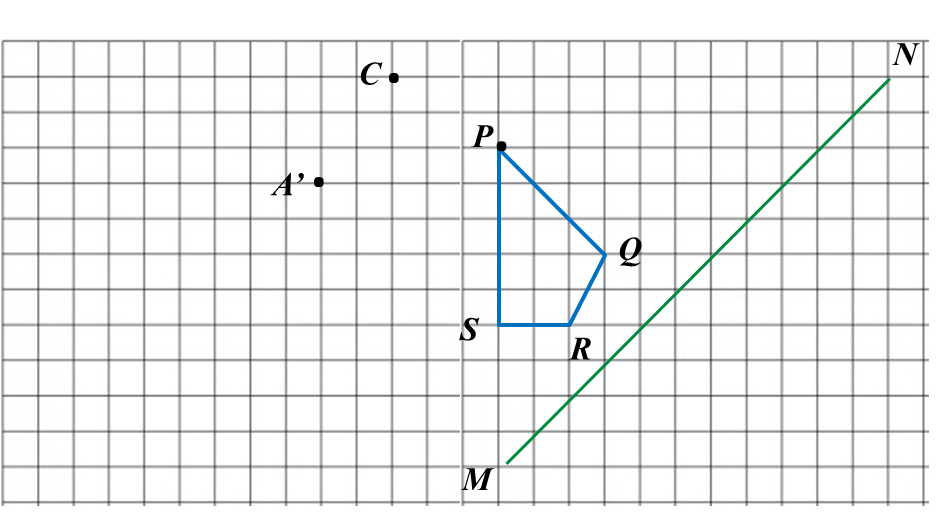Solution:
(a) A’ is the image of P under a rotation of 90o clockwise about the centre C.

(b) and (c)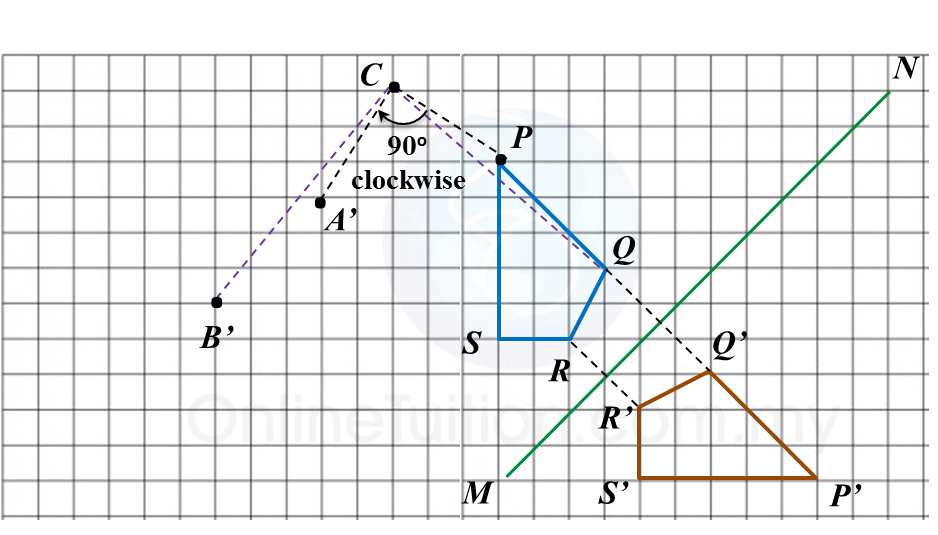Question 8:
Diagram in the answer space shows trapezium ABCD drawn on a Cartesian plane. AD’ is the image of AD under a rotation at centre W.
(a) State
(i) angle of rotation
(ii) the direction of the rotation.
(b) On diagram in the answer space, complete the image of trapezium ABCD.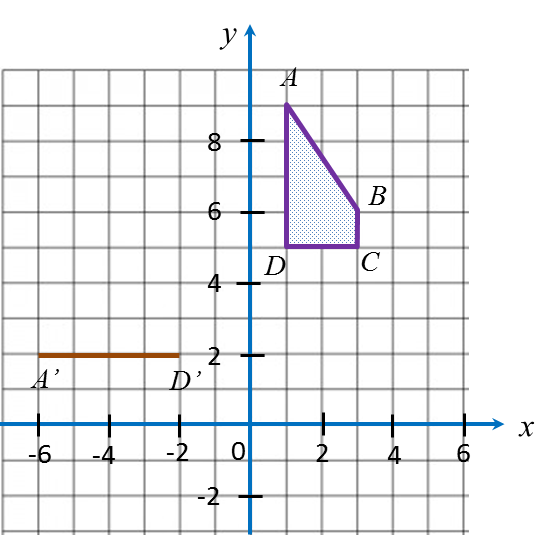Solution:
(a)(i)

(a)(ii) Anticlockwise or clockwise.

(b)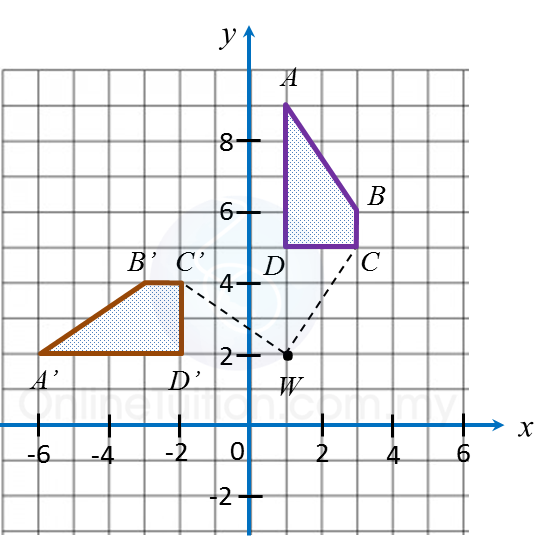# 11.2.1 Transformations (I), PT3 Focus Practice

11.2.1 Transformations (I), PT3 Focus Practice

Question 1:
Diagram below in the answer space shows object drawn on a grid of equal squares with sides of 1 unit.
On the diagram, draw the image of object under the translation $\left(\begin{array}{l}-6\\ \text{}3\end{array}\right).$

:Solution
: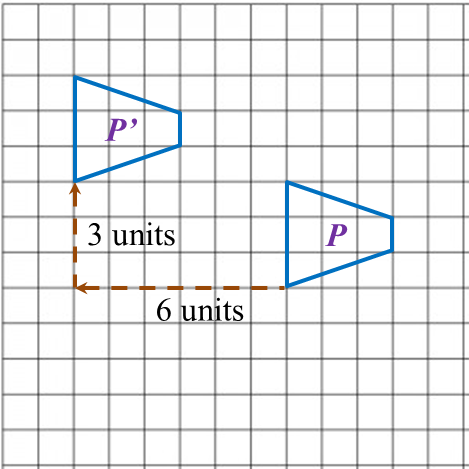Question 2:
Describe the translation which maps point P onto point P’.Solution
: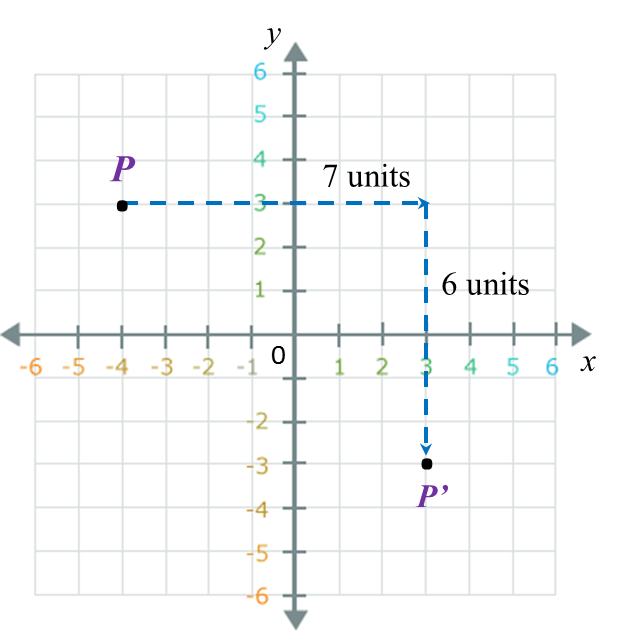The translation is $\left(\begin{array}{l}\text{}7\\ -6\end{array}\right).$

Question 3:
Diagram below in the answer space shows quadrilateral PQRS. R’S’ is the image of RS under a reflection in the straight line AB.
On diagram in the answer space, complete the image of quadrilateral PQRS.

: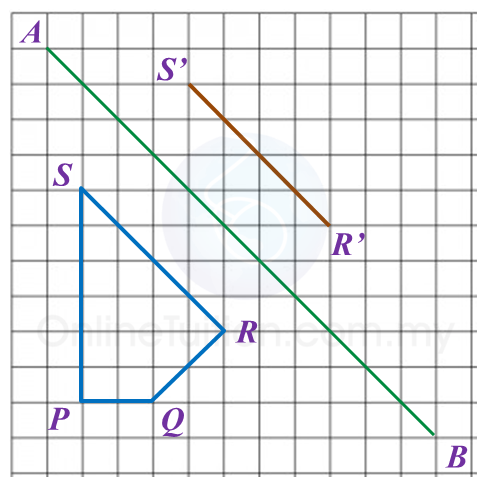Solution:Question 4:
Diagram in the answer space, shows two polygons, M and M’, drawn on a grid of equal squares with sides of 1 unit. M’ is the image of M under a reflection.
(a) Draw the axis of reflection.
(b) Mark the image of P under the same reflection.
(c) Draw the image of M under reflection in the x-axis.

:Solution:Question 5:
On diagram in the answer space, triangle P’Q’R’ is the image of triangle PQR under a rotation about centre C.
(a) State the angle and direction of the rotation.
(b) K’is the image of point K under the same rotation.
Mark and state the coordinates of K’.

: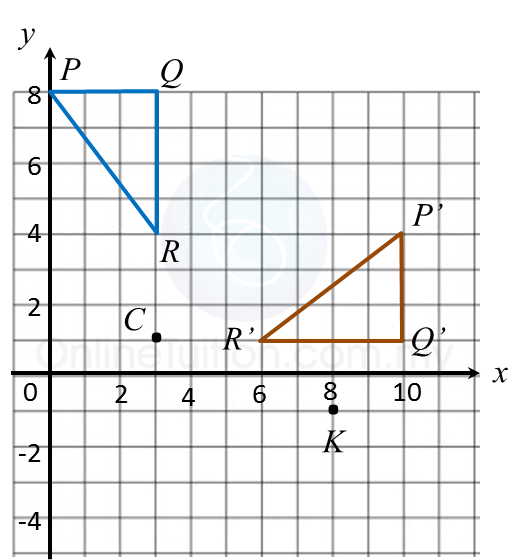Solution: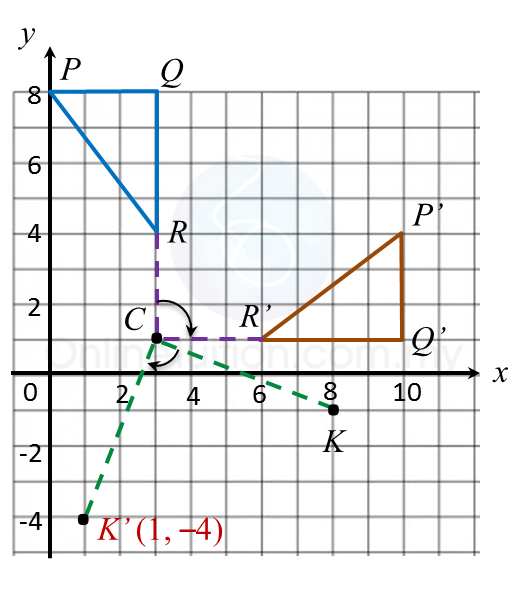(a) ∆ P’Q’R’ is the image of ∆ PQR under a clockwise rotation of 90o.
(b) Image of K = (1, –4).

# 11.1 Transformations (I)

11.1 Transformations (I)

11.1.1 Transformation
A transformation is a one-to-one correspondence or mapping between points of an object and its image on a plane.

11.1.2 Translation
1. A translation is a transformation which moves all the points on a plane through the same distance in the same direction.

2. Under a translation, the shape, size and orientation of object and its image are the same.

3. A translation in a Cartesian plane can be represented in the form $\left(\begin{array}{l}a\\ b\end{array}\right),$  whereby, a represents the movement to the right or left which is parallel to the x-axis and b represents the movement upwards or downwards which is parallel to the y-axis.

Example 1
:
Write the coordinates of the image of A (–2, 4) under a translation $\left(\begin{array}{l}\text{}4\\ -3\end{array}\right)$  and B (1, –2) under a translation $\left(\begin{array}{l}-5\\ \text{}3\end{array}\right)$ .

Solution
:

A’ = [–2 + 4, 4 + (–3)] = (2, 1)
B’ = [1 + (–5), –2 + 3] = (–4, 1)

Example 2
:
Point moved to point K’ (3, 8) under a translation $\left(\begin{array}{l}-4\\ \text{}3\end{array}\right).$
What are the coordinates of point K?

Solution
:
$K\left(x,\text{}y\right)\to \left(\begin{array}{l}-4\\ \text{}3\end{array}\right)\to K\text{'}\left(3,\text{}8\right)$
The coordinates of K = [3 – (– 4), 8 – 3]
= (7, 5)

Therefore the coordinates of K are (7, 5).

11.1.3 Reflection
1. A reflection is a transformation which reflects all points of a plane in a line called the axis of reflection.
2. In a reflection, there is no change in shape and size but the orientation is changed. Any points on the axis of reflection do not change their positions.

11.1.4 Rotation
1. A rotation is a transformation which rotates all points on a plane about a fixed point known as the centre of rotation through a given angle in a clockwise or anticlockwise direction.
2. In a rotation, the shape, size and orientation remain unchanged.
3. The centre of rotation is the only point that does not change its position.

Example 4
:
Point A (3, –2) is rotated through 90o clockwise to A’ and 180o anticlockwise to A1 respectively about origin.
State the coordinates of the image of point A.

Solution
:
Image A’ = (–2, 3)
Image A= (–3, 2)

11.1.5 Isometry
1. An isometry is a transformation that preserves the shape and size of an object.
2.Translation, reflection and rotation and a combination of it are isometries.

11.1.6 Congruence
1. Congruent figures have the same size and shape regardless of their orientation.
2. The object and the image obtained under an isometry are congruent.

# 10.2.3 Circles I, PT3 Focus Practice

            
Question 11:
  
The Town Council plans to build an equilateral triangle platform in the middle of a roundabout. The diameter of circle RST is 24 m and the perpendicular distance from R to the line ST is 18 m. as shown in Diagram below.Find the perimeter of the platform.
  
  Solution:Given diameter = 24 m
  hence radius = 12 m
  O is the centre of the circle.
  Using Pythagoras’ theorem:
            
  
 
      
Question 12:
 
Amy will place a ball on top of a pillar in Diagram below. Table below shows the diameters of three balls X, and Z.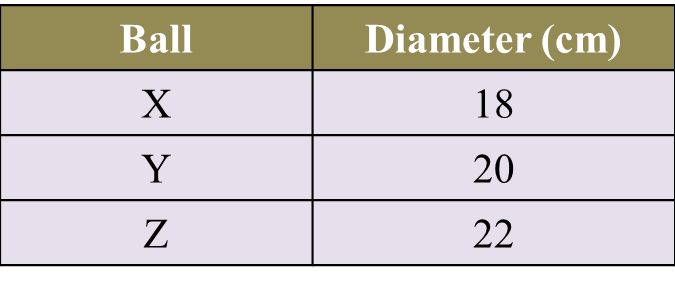Which ball X, or Z, can fit perfectly on the top of the pillar? Show the calculation to support Amy’s choice.
 
 Solution:Question 13:
 
Diagram below shows a rim of a bicycle wheel with a diameter of 26 cm. Kenny intends to build a holder for the rim.Which of the rim holder, X, or Z, can fit the bicycle rim perfectly? Show the calculation to support your answer.
 
 Solution: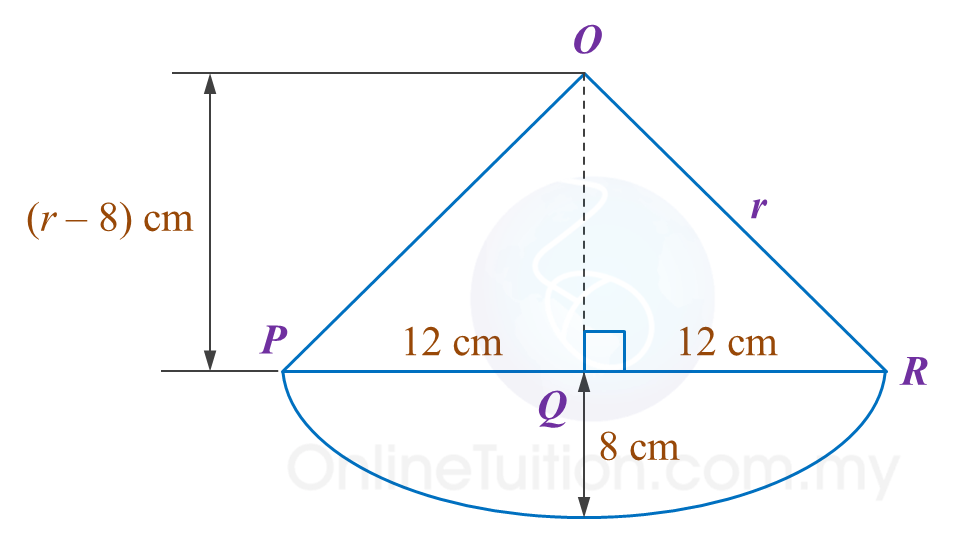# 10.2.2 Circles I, PT3 Focus Practice

                       
Question 6:
       In the diagram below, CD is an arc of a circle with centre O.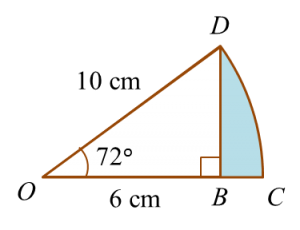Determine the area of the shaded region.
                                                    
 
 Solution:

                                             
      
    
      
                   
 Question 7:
 In diagram below, ABC is a semicircle with centre O.Calculate the area, in cm2 , of the shaded region.
                                     
 
   Solution:
                                         
 
 
                   
 Question 8:
 In diagram below, ABC is an arc of a circle centre O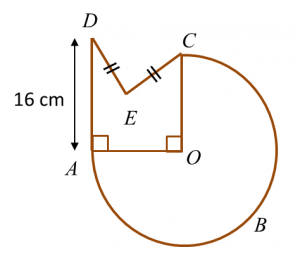The radius of the circle is 14 cm and AD = 2 DE.
   Calculate the perimeter, in cm, of the whole diagram.
                                       
 
    Solution:
                                       
 
 
 [adinserter block="3"]                
Question 9:
 
In diagram below, KLMN is a square and KLON is a quadrant of a circle with centre K.Calculate the area, in cm2, of the coloured region.
                     
 
 Solution:
               
 
 
 
 [adinserter block="3"]                
Question 10:
 
Diagram below shows two quadrants, AOC and EOD with centre O.Sector AOB and sector BOC have the same area.
 Calculate the area, in cm2, of the coloured region.
                     
 
 Solution:
               
 
 
 

# 10.2.1 Circles I, PT3 Focus Practice

10.2.1 Circles I, PT3 Focus Practice

Question 1:
Diagram below shows a circle with centre O.

The radius of the circle is 35 cm.
Calculate the length, in cm, of the major arc AB.

Solution:
Angle of the major arc AB = 360o – 144o= 216o
$\begin{array}{l}\text{Length of major arc}AB\\ =\frac{{216}^{o}}{{360}^{o}}×2\pi r\\ =\frac{{216}^{o}}{{360}^{o}}×2×\frac{22}{7}×35\\ =132\text{cm}\end{array}$

Question 2:
In diagram below, O is the centre of the circle. SPQ and POQ are straight lines.

The length of PO is 8 cm and the length of POQ is 18 cm.
Calculate the length, in cm, of SPT.

Solution
:
Radius = 18 – 8 = 10 cm
PT2 = 102 – 82
= 100 – 64
= 36
PT = 6 cm
Length of SPT = 6 + 6
= 12 cm

Question 3:
Diagram below shows two circles. The bigger circle has a radius of 14 cm with its centre at O.
The smaller circle passes through O and touches the bigger circle.

Calculate the area of the shaded region.

Solution:

Question 4:
Diagram below shows two sectors. ABCD is a quadrant and BED is an arc of a circle with centre C.

Calculate the area of the shaded region, in cm2.

Solution
:

Question 5:
Diagram below shows a square KLMN. KPN is a semicircle with centre O.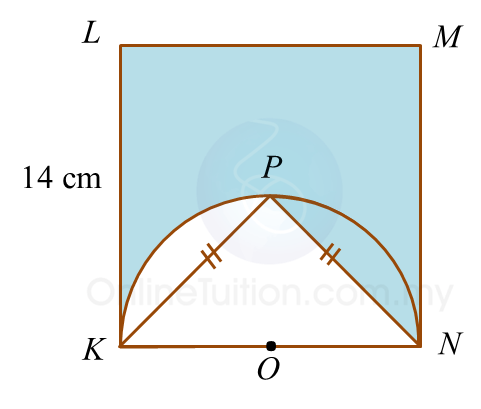Calculate the perimeter, in cm, of the shaded region.

Solution
:

= KL + LM + MN + NP + Arc length PK
= 14 + 14 +14 + 9.90 + 11
= 62.90 cm

# 10.1 Circles I

     
   
10.1 Circles I
   

   
10.1.1 Parts of a Circle
   
1. A circle is set of points in a plane equidistant from a fixed point.
   

   2.
Parts of a circle:
   
(a)    The centre, O, of a circle is a fixed point which is equidistant from all points on the circle.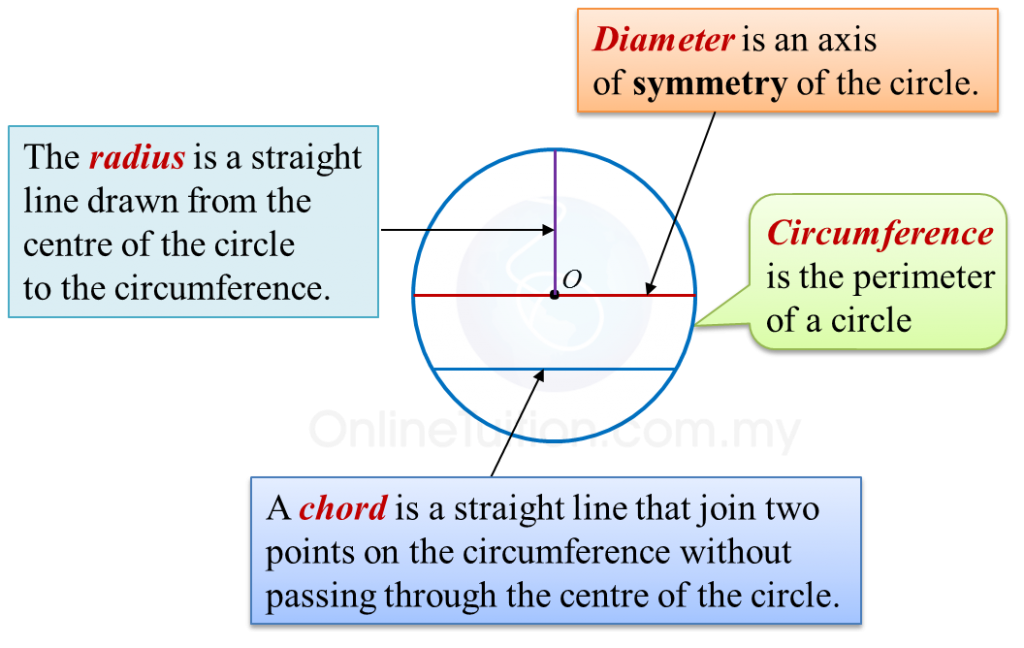(b)
A sector is the region enclosed by two radii and an arc.
   
   
   
       
 
     

   
   (c)
An arc is a part of the circumference of a circle.
       
   

   
   (d)
A segment is an area enclosed by an arc and a chord.
   

   
   
       
 
 
     
   
10.1.2 Circumference of a Circle
   
           
     
Example:
   
Calculate the circumference of a circle with a diameter of 14 cm.     $\left(\pi =\frac{22}{7}\right)$    
   
   

    Solution
:
      $\begin{array}{l}\text{Circumference}=\pi ×\text{Diameter}\\ \text{}=\frac{22}{7}×14\\ \text{}=44\text{cm}\end{array}$

   

   
 
     
   
10.1.3 Arc of a Circle
   
The length of an arc of a circle is proportional to the angle at the centre.
           
     
Example:
   
       

   
Calculate the length of the minor arc AB of the circle above.     $\left(\pi =\frac{22}{7}\right)$    
   

    Solution
:
   
    $\begin{array}{l}\frac{\text{Length of arc}}{\text{Circumference}}=\frac{\text{Angle at centre}}{{360}^{o}}\\ \\ \text{Length of arc}AB=\frac{{120}^{o}}{{360}^{o}}×2×\frac{22}{7}×7\\ \text{}=14\frac{2}{3}\text{cm}\end{array}$

   
   
 
 
     
   
10.1.4 Area of a Circle
   
   
   
   
         
   

   
Example:
   
Calculate the area of each of the following circles that has
   
(a) a radius of 7 cm,
   
(b)   a diameter of 10 cm.
   
    $\left(\pi =\frac{22}{7}\right)$    
   

    Solution
:
   
(a)
   
    $\begin{array}{l}\text{Area of a circle}=\pi {r}^{2}\\ \text{}=\frac{22}{\overline{)7}}×\overline{)7}×7\\ \text{}=154{\text{cm}}^{2}\end{array}$    
 
     
(b)
   
    $\begin{array}{l}\text{Diameter of circle}=10\text{cm}\\ \text{Radius of circle}=5\text{cm}\\ \text{Area of circle}=\pi {r}^{2}\\ \text{}=\frac{22}{7}×5×5\\ \text{}=78.57{\text{cm}}^{2}\end{array}$

   
 
 
     
   
10.1.5 Area of a Sector
   
The area of a sector of a circle is proportional to the angle at the centre.
   
         
   

    Example
:
       

   
   

   
   
   
   
   
        $\begin{array}{l}\text{Area of sector}ABC\\ =\frac{{72}^{o}}{{360}^{o}}×\frac{22}{7}×7×7\\ =30\frac{4}{5}{\text{cm}}^{2}\end{array}$